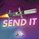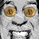8673 lượt xem
Autocorrelation, also known as serial correlation, is the correlation of a signal with a delayed copy of itself as a function of delay.

This indicator displays autocorrelation based on lag number. The autocorrelation is not displayed based on time on the x-axis. It's based on the lag number which is from 1 to 50. The calculations can be done with "Log Returns", "Absolute Log Returns" or "Squared Log Returns". An alternative to use any source as input is also available.

One commonly used method to calculate autocorrelation is to use the "correlation() function". However, Autocorrelation requires one mean and that's the mean of the sample without lag. Since using the correlation function would result in two different means, one from the current sample and one from the lagged sample, to be taken into account it results in incorrect values, albeit the difference being potentially relatively small. Also, the value used as mean needs to remain unchanged regardless of lag number, which also wouldn’t be the case if the correlation() function is utilized in the calculation.

When calculating autocorrelation, the resulting value will range from +1 to -1, in line with the traditional correlation statistic. An autocorrelation of +1 represents a perfect correlation (an increase seen in one time series leads to a proportionate increase in the other time series). An autocorrelation of -1, on the other hand, represents a perfect inverse correlation (an increase seen in one time series results in a proportionate decrease in the other time series).

Lag number indicates which historical data point is autocorrelated. For examples, if lag 3 shows significant autocorrelation, it means current data is influenced by the data three bars ago.

There is "confidence interval" to determine if autocorrelation is statistically significant. The default confidence interval is 1.96 standard deviation which is 95% confidence. The values that surpasses the "critical levels" is significant.

Any value of autocorrelation from lag 1 to 50 that is significantly above or below the "confidence interval threshold", is evidence of either "trend/momentum (when positive)" or "cycle/mean reversion (when negative)".

Absolute returns or squared returns are ways to measure volatility . There is usually significant positive autocorrelation in absolute returns or squared returns. We will often see an exponential decay of autocorrelation in volatility . This means that current volatility is dependent on historical volatility and the effect slowly dies off as the lag increases. This effect shows the property of "volatility clustering". Which means large changes tend to be followed by large changes, of either sign, and small changes tend to be followed by small changes. The autocorrelation effect is more significant in absolute returns than in squared returns.

When most of the autocorrelation from 1 to 50 is within the confidence interval, the returns are likely to be random or IID (independent and identically distributed). Autocorrelation in price is always significantly positive and has an exponential decay. This predictably positive and relatively large value makes the autocorrelation of price (not returns) generally less useful.

Autocorrelation function is also used in time series analysis such as fitting an ARIMA model. It can be used with PACF (Partial Autocorrelation Function) to determine whether to use AR or MA model (Auto Regressive & Moving Average processes). It can also be used to identify the numbers of AR or MA terms.

The chart displays the autocorrelation of "log returns" then the autocorrelation of "squared log returns" below it and at the bottom the autocorrelation of "price".

The "Lag Panel" allows you to navigate the bars and view the value of each lag separately. The lag number to view can be entered in settings. To adjust the thickness of each bar displayed change the histogram width in settings. The minimum period in the setting is 51 because we display 50 lags of autocorrelation on the chart.

Note: conventional "Expected Move", "Probability Cone" and "Probability Panel" are calculated with the assumption of IID (which assumes no significant autocorrelation). Therefore, when there is significant positive autocorrelation, they would underestimate the volatility , and when there is significant negative autocorrelation they would overestimate volatility . Using ACF we can see the significance of autocorrelation that might exist and decide whether or not to use "scale with autocorrelation" function in our "probability indicators" (to adjust for the effect of autocorrelation on the model).
Phát hành các Ghi chú: Added functionality to make the lag panel more visible. Also, changed the chart to autocorrelation of absolute returns to better showcase the exponential decay.
Phát hành các Ghi chú: updated chart to display autocorrelation of log returns , absolute returns and price.
Mã nguồn mở

Với tinh thần của TradingView, tác giả đã xuất bản tập lệnh theo mã nguồn mở, vì thế trader có thể dễ dàng hiểu và tùy chỉnh được. Bạn có thể sử dụng miễn phí, hoặc tùy chỉnh lại mã đã được cấp phép bởi Quy tắc Chung. Bạn có thể sử dụng nó trên biểu đồ.

Bạn muốn sử dụng tập lệnh này trên biểu đồ?
Our Website🅱🅰🐷: https://bapigindicator.com

https://discord.gg/kCJYghDZcd

## Bình luậnI still admire this indicators birth with it's vivid color, code elegance, and stylized data presentation. Keep it up! Happy New Year!! ♥
Phản hồiThis publication will be featured in our "Best Scripts of The Month" selection. Thank you for your valuable contribution to the TradingView community, and congrats!
Phản hồiThanks guys, this is phenomenal work. You're just churning out next level s**t like a factory right now. Been getting to grips theoretically with autocorrellation/time series for while now, so can't wait to play with this to get a deeper understanding... Thanks a lot! MrD
Phản hồiDamn you guys are mental giants, thanks very much for the hard work!!!
Phản hồi